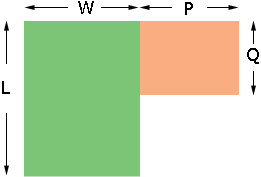Name: Michael Who is asking: Student Level of the question: All Question: Dear Sir, I would like to know how to measure the area of a house? Like is it measure length x breath x height=area? Thank you Hi Michael, If the house is on a rectangular base then the area is the length times the width, but most houses aren't rectangular. Suppose that the base of the house is as in the diagram.Then you need to divide the house into two pieces and find the area of each piece individually.The area of the green section is its length (L) times its width (W) and that area of the orange section is its length (P) times its width (Q). Thus the area of the house is (LW) + (PQ) Penny